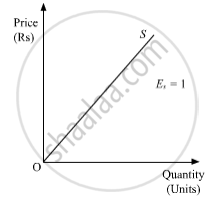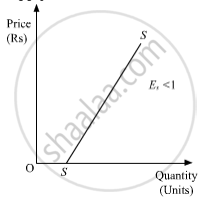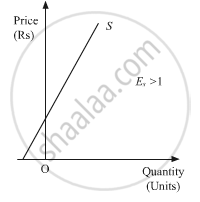Advertisement Remove all ads

# Answer the Following About 200 to 250 Words Explain Various Methods to Measure Price Elasticity of Supply. - Economics

Answer in Brief

Answer the following about 200 to 250 words
Explain various methods to measure Price Elasticity of Supply.

Advertisement Remove all ads

#### Solution

The price elasticity of supply can be measured by the following two methods namely:

1. Proportionate Method

2. Geometric Method

Proportionate or percentage method

According to this method, the price elasticity of supply is given, by the ratio of percentage change in the quantity supplied and percentage change in the price of commodity.

Algebraically, it can be expressed as:

Es = "Percentage Change in Quantity Supplied"/"Percentage Change in Price"

Geometric method

The geometric method is a graphical presentation of the elasticity of the supply. This method does not involve any calculation. Just by looking at the shape of the supply curve, we can infer the degree of the elasticity of the supply. The degree of the price elasticity of supply depends on the slope and origin position of the supply curve.

There are following possible situations.

a. Unitary Elasticity of Supply (Es=1 )- If the straight line supply curve originates from the origin, then irrespective of the angle of inclination of the supply curve, the elasticity of supply will always be equal to one i.e. (Es=1 ). Such a supply curve is called unitary elastic supply curve.b. Less Elastic Supply (Es < 1)- If the supply curve originates from the horizontal intercept of quantity-axis, then irrespective of the angle of inclination of the supply curve, the elasticity of the supply curve will be less than one i.e. (Es < 1)c. More Elastic Supply (Es > 1)- Unlike the less elastic supply curve, the relatively more elastic supply curve originates from the vertical intercept of price-axis. The value of elasticity of supply originating from the vertical intercept is greater than one, i.e. (Es > 1)Is there an error in this question or solution?
Advertisement Remove all ads

#### APPEARS IN

Micheal Vaz Economics HSC 12th Standard Maharashtra State Board
Chapter 5 Producer's Behaviour
Exercise | Q 4 | Page 45
Advertisement Remove all ads

#### Video TutorialsVIEW ALL 

Advertisement Remove all ads
Share
Notifications

View all notifications

Forgot password?